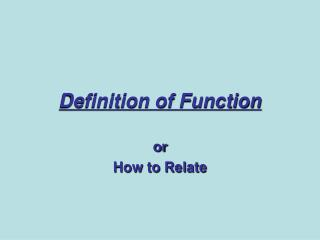DownloadDownload PresentationDefinition of Function

# Definition of Function

Télécharger la présentation## Definition of Function

- - - - - - - - - - - - - - - - - - - - - - - - - - - E N D - - - - - - - - - - - - - - - - - - - - - - - - - - -
##### Presentation Transcript

1. Definition of Function or How to Relate

2. Definition of a Relation

3. Relation (A) (B) • 32 mpg • 8 mpg • 16 mpg (C)

4. Domain and Range • The values that make up the set of independent values are the domain • The values that make up the set of dependent values are the range. • State the domain and range from the 4 examples of relations given.

5. CorrespondenceorRelation Domain Range

6. Definition of a Relation • A Relation maps a value from the domain to the range. A Relation is a set of ordered pairs. • The most common types of relations in algebra map subsets of real numbers to other subsets of real numbers.

7. Example

8. Definition of a Function • If a relation has the additional characteristic that each element of the domain is mapped to one and only one element of the range then we call the relation a Function.

9. Definition of a Function • If we think of the domain as the set of boys and the range the set of girls, then a function is a monogamous relationship from the domain to the range. Each boy gets to go out with one and only one girl. • But… It does not say anything about the girls. They get to live in Utah.

10. Decide if the Relation is a Function. • The relation is the year and the cost of a first class stamp. • The relation is the weight of an animal and the beats per minute of it’s heart. • The relation is the time of the day and the intensity of the sun light. • The relation is a number and it’s square. • The relation is time since you left your house for work and your distance from home.

11. Examples Please • Give three examples from the real world of relations. Be sure and state the domain, the range, and the definition of how the variables are related. • Decide which if any of your examples are functions.

12. y1 y2 RANGE NOT A FUNCTION R x DOMAIN

13. x1 y x2 RANGE DOMAIN FUNCTION f

14. X Y 1 1 -5 1 -1 1 3 1 X Y 1 2 -5 7 -1 2 3 3 X Y 1 2 1 7 1 2 1 3 X Y 1 π π 1 -1 5 π 3 Mathematical Examples • Decide if the following relations are functions.

15. X Y 1 2 5 10 -1 -2 3 6 Ways to Represent a Function • Graphical • Symbolic • Numeric • VerbalThe cost is twice the original amount.

16. Function NotationThe Symbolic Form • A truly excellent notation. It is concise and useful.

17. Name of the function • Output Value• Member of the Range• Dependent Variable These are all equivalent names for the y. • Input Value• Member of the Domain• Independent Variable These are all equivalent names for the x.

18. Examples of Function Notation • The f notation • Find f(2), g(-1), f(-0.983),

19. Your Turn! Given: Evaluate the following:

20. Graphical Representation • Graphical representation of functions have the advantage of conveying lots of information in a compact form. There are many types and styles of graphs but in algebra we concentrate on graphs in the rectangular (Cartesian) coordinate system.

21. Range Domain Graphs and Functions

22. Vertical Line Test for Functions • If a vertical line intersects a graph once and only once for each element of the domain, then the graph is a function.

23. How to determine Domain and Range of a function. • Graph the following on your calculator. Also give the algebra.

24. Big Deal! • A point is in the set of ordered pairs that make up the function if and only if the point is on the graph of the function.

25. Key Points • Definition of a function • Ways to represent a functionSymbolicallyGraphicallyNumericallyVerbally